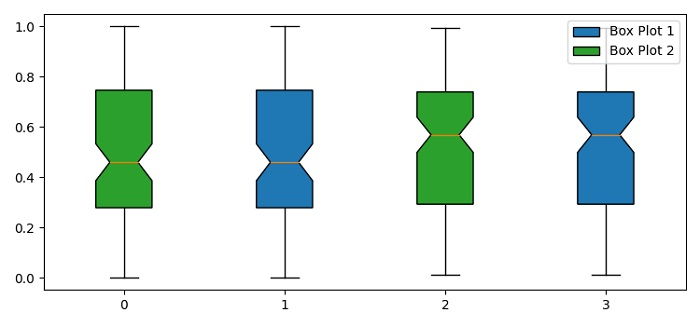# Adding a legend to a Matplotlib boxplot with multiple plots on the same axis

To add a legend to a matplotlib boxplot with multiple plots on the same axis, we can take the following steps −

• Set the figure size and adjust the padding between and around the subplots.

• Create random data, a and b, using numpy.

• Create a new figure or activate an existing figure using figure() method.

• Add an axes to the current figure as a subplot arrangement.

• Make a box and whisker plot using boxplot() method with different facecolors.

• To place the legend, use legend() method with two boxplots, bp1 and bp2, and ordered label for legend elements.

• To display the figure, use show() method.

## Example

import matplotlib.pyplot as plt
import numpy as np

plt.rcParams["figure.figsize"] = [7.50, 3.50]
plt.rcParams["figure.autolayout"] = True

a = np.random.rand(100, 2)
b = np.random.rand(100, 2)

fig = plt.figure()
plt.show()